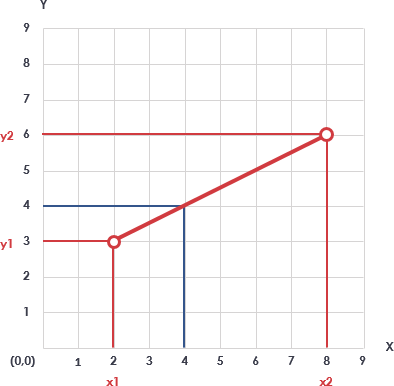# Interpolation Calculator

Interpolation is a useful mathematical and statistical tool used to find a value between two points on a line or curve. If linear interpolation formula is concerned then it can be used to find the new value from the two given points. If we compare it to Lagrange’s interpolation formula, the “n” set of numbers is needed.

## Linear Interpolation Example

Linear interpolation involves estimating a new value by connecting two adjacent known values with a straight line. If the two known values are (x1, y1) and (x2, y2), then the y value for some point x is. Suppose:

X1 = 2, Y1 = 3

X2 = 8, Y2 = 6

Target X = 4`y - y1 = [(y2 - y1) / (x2 - x1)] * (x - x1)`

Where,

• x1 and y1 are the first coordinates
• x2 and y2 are the second coordinates
• x is the point to perform the interpolation
• y is the interpolated value.

Target Y = 4 as shown in the Figure above.

## Interpolation calculator var vtbempsid = vtbempsid||0;vtbempsid++; (function(w,d,tid,sn,rn,options){ var eid='vtbemps'+vtbempsid; var sns=d.getElementsByTagName('script'); var st=sns[sns.length-1]; var width=st.getAttribute('data-width')||'1017px'; var height=st.getAttribute('data-height')||'539px'; var e=d.createElement('script'); e.type='text/javascript'; e.id=eid; e.src='https://online.visual-paradigm.com/app/tabular/embed.js'; e.onload=function(){VTBEMPS(eid,width,height,tid,sn,rn,options);}; e.onreadystatechange=function(){if (this.readyState==='complete'){VTBEMPS(eid,width,height,tid,sn,rn);}}; st.parentNode.insertBefore(e,st); }) (window,document,'f4a9ada0-45f4-4405-a54c-2d0672e19164','','','scrollbars:no')

#### This calculator is created with

the best online spreadsheet editor with excellent formula and editing capability.

For more calculators for finance, mathematics health, unit converters and more check out our calculators collection.